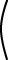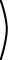Is there another N×N matrix that works like I?

No. This is not quite obvious, but since 1 is unique for real numbers you might suspect that I is unique for NxN matrices.

# I is Unique

Here is why this is so. In the following, assume that all the matrices have the correct dimensions for the multiplications. Suppose there is a matrix Z and that

ZA = A           (1)

for any A with the right dimensions. The matrix Z works like I, but we are hoping that it will be different. We know how I works:

BI = B           (2)

for any B with the right dimensions. Now replace A with I in (1) (since A can be any suitable matrix):

ZI = I           (3)

Now replace B with Z in (2):

ZI = Z           (4)

Looking at (3) and (4), Z and I are both equal to the same thing, so they must equal each other:

Z = I           (5)

You can start at step (1) with the other order AZ = A and reach the same conclusion. So if you have found a matrix that works like the indentity, it is indeed the identity matrix.

### QUESTION 5:

What is the transpose of I?```1 0 0 0 1 0 0 0 1 ```T